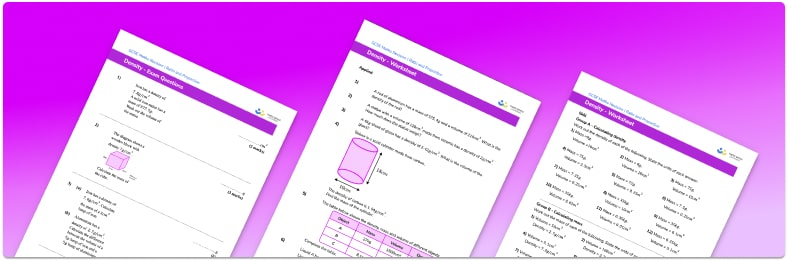# Density Worksheet• Section 1 of the density worksheet contains 20+ skills-based density practice questions, in 3 groups to support differentiation
• Section 2 contains 3 applied density questions with a mix of worded problems and deeper problem solving questions
• Section 3 contains 3 foundation and higher level GCSE exam style questions on density questions
• Answers and a mark scheme for all density questions are provided
• Questions follow variation theory with plenty of opportunities for students to work independently at their own level
• All questions created by fully qualified expert secondary maths teachers
• Suitable for GCSE maths revision for AQA, OCR and Edexcel exam boards

• This field is for validation purposes and should be left unchanged.

You can unsubscribe at any time (each email we send will contain an easy way to unsubscribe). To find out more about how we use your data, see our privacy policy.

### Density at a glance

Density is a measure of the mass of something per unit volume. It is usually measured in kilograms per cubic metre or grams per cubic centimetre. Calculating density is done using the density formula: Density = Mass divided by Volume.

For example, if we have a piece of wood with a volume of 100cm3 and a mass of 80g, we can calculate the density of the object: Density = 80 divided by 100 = 0.8g/cm^3. Sometimes we might be given information about the side lengths of the object rather than the volume, in which case we start by calculating the volume and then use this for the density calculation

By rearranging the density formula, we can get the following formulae for volume and mass:

Volume = Mass divided by Density and Mass = Density multiplied by Volume. The answers we get by using these formulae may be non-exact, in which case we can round them to an appropriate number of decimal places or significant figures

Some useful conversions include: 1 ml of water fills 1cm^3, and 1000kg = 1 tonne. We therefore multiply millilitres by 1 to get centimetres, and we divide kilograms by 1000 to get tonnes.

Looking forward, students can then progress to additional exchange and conversion rates worksheets, and other ratio and proportion worksheets for example a ratio worksheet or aFor more teaching and learning support on Ratio and Proportion our GCSE maths lessons provide step by step support for all GCSE maths concepts.

## Do you have KS4 students who need more focused attention to succeed at GCSE?There will be students in your class who require individual attention to help them succeed in their maths GCSEs. In a class of 30, it’s not always easy to provide.

Help your students feel confident with exam-style questions and the strategies they’ll need to answer them correctly with our dedicated GCSE maths revision programme.

Lessons are selected to provide support where each student needs it most, and specially-trained GCSE maths tutors adapt the pitch and pace of each lesson. This ensures a personalised revision programme that raises grades and boosts confidence.

Find out more